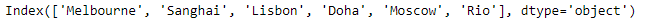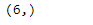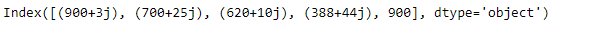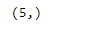# Python | Pandas Index.shape

Pandas Index is an immutable ndarray implementing an ordered, sliceable set. It is the basic object which stores the axis labels for all pandas objects.

Pandas` Index.shape` attribute return a tuple of the shape of the underlying data in the given Index object.

Syntax: Index.shape

Parameter : None

Returns : tuple

Example #1: Use `Index.shape` attribute to return a tuple of the shape of the underlying data in the given Index object.

 `# importing pandas as pd ` `import` `pandas as pd ` ` `  `# Creating the index ` `idx ``=` `pd.Index([``'Melbourne'``, ``'Sanghai'``, ``'Lisbon'``, ``'Doha'``, ``'Moscow'``, ``'Rio'``]) ` ` `  `# Print the index ` `print``(idx) `

Output :Now we will use `Index.shape` attribute to return a tuple of the shape of the underlying data in the given Index object.

 `# return a tuple of the shape ` `# of data in idx object ` `result ``=` `idx.shape ` ` `  `# Print the result ` `print``(result) `

Output :As we can see in the output, the `Index.shape` attribute has returned a tuple based on the size of the underlying data in the given Index object.

Example #2 : Use `Index.shape` attribute to return a tuple of the shape of the underlying data in the given Index object.

 `# importing pandas as pd ` `import` `pandas as pd ` ` `  `# Creating the index ` `idx ``=` `pd.Index([``900` `+` `3j``, ``700` `+` `25j``, ``620` `+` `10j``, ``388` `+` `44j``, ``900``]) ` ` `  `# Print the index ` `print``(idx) `

Output :Now we will use `Index.shape` attribute to return a tuple of the shape of the underlying data in the given Index object.

 `# return a tuple of the shape ` `# of data in idx object ` `result ``=` `idx.shape ` ` `  `# Print the result ` `print``(result) `

Output :As we can see in the output, the `Index.shape` attribute has returned a tuple based on the size of the underlying data in the given Index object.

My Personal Notes arrow_drop_upCheck out this Author's contributed articles.

If you like GeeksforGeeks and would like to contribute, you can also write an article using contribute.geeksforgeeks.org or mail your article to contribute@geeksforgeeks.org. See your article appearing on the GeeksforGeeks main page and help other Geeks.

Please Improve this article if you find anything incorrect by clicking on the "Improve Article" button below.

Article Tags :

Be the First to upvote.

Please write to us at contribute@geeksforgeeks.org to report any issue with the above content.